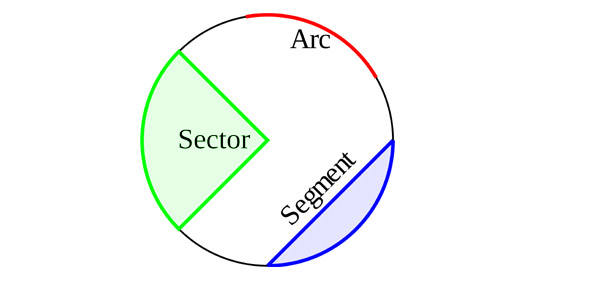16 Questions | Total Attempts: 426SettingsA circle is a closed two-dimensional figure in which the set of all the points in the plane is equidistant from a given point called “centre”. Every line that passes through the circle forms the line of reflection symmetry. Also, it has rotational symmetry around the centre for every angle. Know more about circle's vocabulary in this quiz.

• 1.
____- The set of all points in a plane, at a given distance from a given point in the plane.
• 2.
____- Circles with the same radius.
• 3.
____- Circles that lie in the same plane that share the same center.
• 4.
____- A chord that contains the center of the circle.
• 5.
____- A segment from a point on a circle, or a sphere, to the center.
• 6.
____- Distance around a circle. This is the perimeter of the circle.
• 7.
____- An arc of a circle whose endpoints are the endpoints of a diameter.
• 8.
____- Two points on a circle, and the continuous part of the circle, between the two points.
• 9.
____- A fraction of the circumference of a circle defined by the arc.
• 10.
____- An arc of a circle whose length is greater than the length of a semicircle of the circle.
• 11.
____- An arc of a circle whose length is less than the length of a semicircle of the circle.
• 12.
____- A line that lies in the plane of a circle and that intersects the circle at exactly one point.)
• 13.
____- A segment whose end points lie on a circle.
• 14.
____- A line that contains a chord.
• 15.
____- Angle whose vertex is the center of a circle and whose sides contain radii of a circle.
• 16.
____- Angle whose vertex lies on a circle and whose sides contain chords of the circle.
Related TopicsBack to top